# Graphing the Cosecant, Secant & Cotangent Functions

Lesson Transcript
Instructor: Yuanxin (Amy) Yang Alcocer

Amy has a master's degree in secondary education and has been teaching math for over 9 years. Amy has worked with students at all levels from those with special needs to those that are gifted.

The cosecant acts as the reciprocal of the sine, the secant is the reciprocal of the cosine, and the cotangent is the reciprocal of the tangent function. Learn how these appear on graphs, and examine the shifting methods using transformations. Updated: 10/27/2021

## Cosecant Graph

In this video lesson, we talk about the graphs of the three other trigonometric functions of cosecant, secant, and cotangent.

Remember our basic three trigonometric functions are sine, cosine, and tangent. The three functions that we are talking about today are defined as the reciprocals of our basic three functions. So, we have cosecant (csc) is the reciprocal of sine or 1/sine, secant (sec) is the reciprocal of cosine or 1/cosine, and cotangent (cot) is the reciprocal of the tangent function or 1/tangent.

How can you remember these? Someone once told me about the phrase 'Co-Co No Go.' This helped me a lot! This helps me to remember that the cosecant goes with the sine and not the cosine. The tangent and cotangent are easy to remember since they share the same word 'tangent.'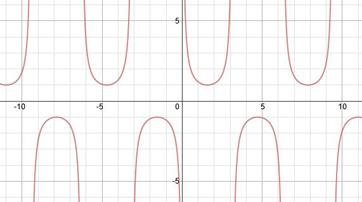Wow, this is interesting. We get mounds on the bottom and dips on the top. Well, this is to be expected. Since this is the reciprocal of the sine function and our denominator is now the sine function, we know that we will have an asymptote whenever the denominator, the sine function, equals 0. So, we have asymptotes at pi*n = 0, pi, 2pi, .... Also as expected, just like the sine function, we have the same standard period, or length of a cycle, of 2pi.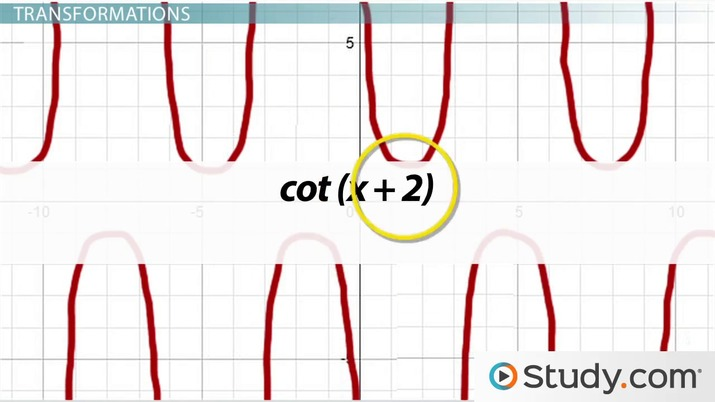An error occurred trying to load this video.

Try refreshing the page, or contact customer support.

Coming up next: Using Graphs to Determine Trigonometric Identity

### You're on a roll. Keep up the good work!

Replay
Your next lesson will play in 10 seconds
• 0:01 Cosecant Graph
• 1:43 Secant Graph
• 2:24 Cotangent Graph
• 2:58 Transformations
• 5:09 Lesson Summary
Save Save

Want to watch this again later?

Timeline
Autoplay
Autoplay
Speed Speed

## Secant Graph

Moving on to the secant graph.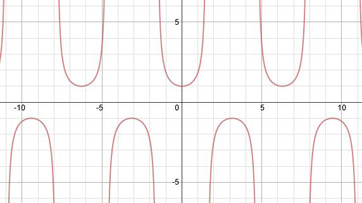Hey, this looks a lot like our cosecant graph! And if we weren't careful we might mistake it for it. We have the same mounds and dips. The only difference is that these mounds and dips occur at different spots now. Just like our cosecant graph, our secant graph has asymptotes wherever our cosine function is 0, so we have asymptotes at (pi/2) + pi*n = pi/2, 3pi/2, 5pi/2, .... Also, because our cosine function has a standard period of 2pi, so does our secant graph.

## Cotangent Graph

Lastly, here is our cotangent graph: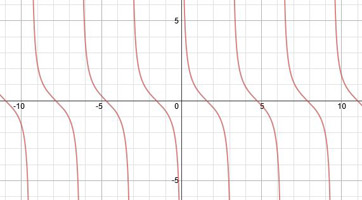Just like the other two graphs, our cotangent graph has asymptotes wherever our tangent function is equal to 0. Our tangent function equals 0 every pi*n spaces, so at 0, pi, 2pi, and so on. We see that our cotangent function does have asymptotes corresponding to these places. We also see that, just like our tangent function has a standard period of pi, so does our cotangent function.

## Transformations

We've covered all our graphs now. Let's talk about shifting our graphs, or transformations. We can easily make our graph move up, down, to the left and right by just adding or subtracting numbers from different areas of our function.

To move our graph up or down, we can simply add or subtract numbers from the end of our function. For example, the function csc (x) + 2 has a shift of 2 spaces up. If we wanted to move the graph down, we would subtract two from the end.

If we wanted to move our graph to the right, we would subtract numbers from our argument, our variable. So, the graph of cot (x - 2) is the graph of cot (x) shifted 2 spaces to the right. If we add 2 instead of subtracting, we would then have a shift of 2 spaces to the left

If we multiply our function by a number, it will change how wide the middle part is. For example, in the cosecant and secant function, this number will change how far apart the mounds are to the dips. Larger numbers make the mounds go lower and the dips higher thereby increasing the distance between the two. So, the graph of 4 csc (x) looks like this: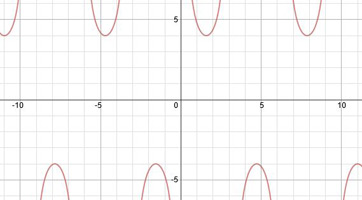To unlock this lesson you must be a Study.com Member.

## Practice:Graphing the Cosecant, Secant & Cotangent Functions Quiz

1/5 completed

### The secant function has how long of a period?

Create Your Account To Take This Quiz

As a member, you'll also get unlimited access to over 84,000 lessons in math, English, science, history, and more. Plus, get practice tests, quizzes, and personalized coaching to help you succeed.

It only takes a few minutes to setup and you can cancel any time.

### Register to view this lesson

Are you a student or a teacher?